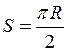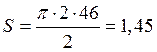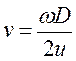# Snake-like, crawling technological robot for the operation inside pipelines. The robot for operation in pipes РТ02/05-1, страница 3

b - width of a wheel, m

lk - interaxal distance between wheels приводного a part, m

l - distance between axes of hinges of parts, m

Lрасч - settlement length of the robot, m

L - length of the robot, m

nрасч - settlement quantity{amount} of parts of the robot

n - quantity{amount} of parts of the robot

d - diameter of the cylinder of a clip of wheels, m

p - pressure in cylinders of a clip of wheels, Па

P - force of a clip of wheels, Н

f - factor of a sliding friction of pair a wheel-pipe

r - radius of friction качения pairs a wheel-pipe, m

s - contact pressure upon a paint from a wheel, Па/м2

[s] - supposed contact pressure upon a paint, Па/м2

w - rated speed of a shaft of the engine of a drive of wheels, with-1

Mдврасч - the settlement twisting moment of a shaft of the engine of a drive of wheels, Nanometer

Мдв - the twisting moment of a shaft of the engine of a drive of wheels, Nanometer

u - transfer number of a reducer of a drive of wheels

h - EFFICIENCY of the mechanism of a drive of wheels

v - nominal linear speed of the robot, km/s

T - useful traction effort of the robot, Н

Tзв - useful traction effort of one приводного a part, Н

3.    The Design procedure

As a result of calculation design data{parameters} of the robot, proceeding from following requirements are defined{determined}:

3.1.  The Center-to-center distance between sprockets of drive links should be such that links were torn in a pipe of minimum diameter at the peak corner{coal} of curving.

3.2.  The Length of the robot and, accordingly, amount of links should be such that at a pipe of the peak diameter and the peak corner{coal} of curving even one link was in a rectilinear part of a pipe.

3.3.  Proceeding from the requirement of axial stiffness of the robot, the relation of amounts drive and intermediates should be such that between any with two drive links was no more than one intermediate link{intermediate}.

3.4.  Parameters of a drive unit should ensure demanded nominal{rated} linear velocity of the robot.

3.5.  At transiting curving a pipe at the peak corner{coal} of curving the link which is being a rectilinear part of a pipe, ensures 80 % of the useful tractive power. This requirement is gained experimental by.

3.6.  Force of a hold-down tool of sprockets to a pipe should be such to not exceed an acceptable value of contact pressure on colour.

3.7.  The Force of sliding friction of pairs of a sprocket-pipe should exceed the useful tractive power, i.e. sprockets should not revolve.

3.8.  The Torque of the drive should ensure driving of the robot, i.e. overcome the useful tractive power and a rolling friction of pairs of a sprocket-pipe.

Thus, from a reason of minimum cost of the robot (drives of a drive unit and blocks of control make a main body of cost of the robot), drives of an amplifying underpower since cost of the drive essentially depends on its{his} power should be selected.

4.    Geometrical calculation

4.1.  By means of computer modelling movement of the robot in a pipe of the minimal diameter the interaxal distance between wheels приводного a part lk and distance between axes of hinges l (a condition 3.1) is certain.

lk = 0,2 m;

l = 0,25 m.

4.2.  Length изогнутого a site of a pipe of the maximal diameter at the maximal corner{coal} of a bend:, Where

R - radius of a bend of a pipe, R=2dтрмах, where

dтрмах - diameter of a pipe of the maximal size, dтрмах=0,5 m.m.

Proceeding from a condition 3.2 settlement length of the robot:

Lрасч=S+l;

Lрасч=1,45+0,25=1,70 .

Then;

nрасч = 1,7/0,25 = 6,8 m.

We accept n = 7.

Then L = 0,25×7 = 1,75 m.

Proceeding from a condition 3.3, we accept quantity{amount} приводных the parts, equal 4, and the quantity{amount} of intermediate parts equal 3.

5.    The Kinematic calculation

Proceeding from a condition 3.4, parameters of the robot are connected by a parity{ratio}:,                                                                                                                      (5)

6.    Power{Force} calculation